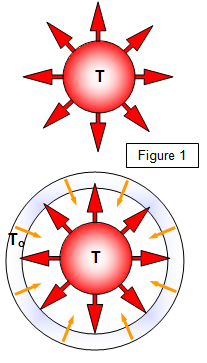# The Stefan-Boltzmann Law

Using apparatus devised by Lummer and Pringsheim the energy emitted by a black body may be measured over a range of different temperatures and in many different regions of the spectrum. The results obtained can be summarised as follows.

(a) the total energy (E) emitted by a black body per unit area per second is proportional to the fourth power of the absolute temperature (T) of the body. This is known as the Stefan-Boltzmann Law.

Energy output per m2 per second (E) = σT4

where σ is a constant known as the Stefan- Boltzmann constant and has a value of 5.7x10-8 Wm2K-4.(b) if the temperature of the body's surroundings are at a temperature To there will be an exchange of heat energy between them and the body. The net loss of energy from the body per unit area becomes:

E = σ[T4 - To4]

(c) for a body of surface area A the net loss of energy per second will be:

E = σA[T4 - To4]

(d) the nature of the surface is allowed for by a quantity known as the emissivity (ε) - for a black body this is equal to 1 and for all other surfaces it is <<1. The equation for the net loss of energy per second now becomes:

E = σεA[T4 - To4]

It is important to realise the difference between this equation and Newton's law of cooling. Stefan's law applies to the loss of energy by radiation while Newton's law applies to loss of energy by convection. Both laws are found to hold for temperature differences of hundreds of degrees.

Example problem
Calculate the heat loss from a space craft of surface area 25 m2 and at a temperature of 300 K if the radiation that it receives from the Sun is equivalent to a temperature in space of 50 K. Assume that the spacecraft behaves as a black body.

Using: E = σA[T4 - To4] = 5.7x10-8x25[3004 - 504] =1.15x104 W = 11.5 kW

WORD VERSION AVAILABLE ON THE SCHOOLPHYSICS CD

© Keith Gibbs 2013